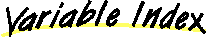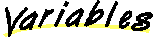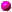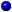`All Packages  Class Hierarchy  This Package  Previous  Next  Index`

# Class logic.Condition

```java.lang.Object
|
+----logic.Condition
```

public class Condition
extends Object
This class abstracts the notion of a "condition" in branching logic. It provides constructors for a variety of simple kinds of conditions such as comparing primitives with =, <, or <=. It also provides a constructor for testing whether objects are equal, and a more general constructor, which accepts a Hashtable, for more complicated conditions.

##b1b2BOOLEANd1d2dataDOUBLEEQUALf1f2FLOATi1i2INTEGERLESSLESS_EQUALob1ob2OBJECTopstatus

##Condition(boolean, boolean)
This constructor compares two booleans to see if they are the same.Condition(double, int, double)
This constructor compares two doubles using the comparison named by oper.Condition(float, int, float)
This constructor compares two float's using the comparison named by oper.Condition(Hashtable)
This version of Condition accepts a Hashtable that must contain all the data that is necessary to implement the evaluate() method -- the evaluate() method must be overridden if this constructor is used.Condition(int, int, int)
This constructor compares two int's using the comparison named by oper.Condition(Object, Object)
This constructor is used when two objects need to be compared.

##badOperator(int)
returns true if the argument op is not one of the legal operatorsevaluate()
If the Hashtable version of the constructor is used, this method must be overridden; otherwise the present version of evaluate should work without modificationevaluateBoolean()evaluateDouble()evaluateFloat()evaluateInteger()evaluateObject()
Note that the equals() method of Object should have been overridden in order for this method to work as desired.getData()

##BOOLEAN
``` final int BOOLEAN
```INTEGER
``` final int INTEGER
```FLOAT
``` final int FLOAT
```DOUBLE
``` final int DOUBLE
```OBJECT
``` final int OBJECT
```status
``` int status
```data
``` Hashtable data
```ob1
``` Object ob1
```ob2
``` Object ob2
```b1
``` boolean b1
```b2
``` boolean b2
```i1
``` int i1
```i2
``` int i2
```f1
``` float f1
```f2
``` float f2
```d1
``` double d1
```d2
``` double d2
```op
``` int op
```LESS
``` public static final int LESS
```LESS_EQUAL
``` public static final int LESS_EQUAL
```EQUAL
``` public static final int EQUAL
```

##Condition
``` public Condition(double d1,
int oper,
double d2) throws IllegalExpressionException
```
This constructor compares two doubles using the comparison named by oper. The default evaluate() method should be used.Condition
``` public Condition(float f1,
int oper,
float f2) throws IllegalExpressionException
```
This constructor compares two float's using the comparison named by oper. The default evaluate() method should be used.Condition
``` public Condition(int i1,
int oper,
int i2) throws IllegalExpressionException
```
This constructor compares two int's using the comparison named by oper. The default evaluate() method should be used.Condition
``` public Condition(Object ob1,
Object ob2)
```
This constructor is used when two objects need to be compared. Usually, the default equals(Object) method provided by Object should be overridden in the class that is the template for the arguments ob1 and ob2.Condition
``` public Condition(Hashtable data)
```
This version of Condition accepts a Hashtable that must contain all the data that is necessary to implement the evaluate() method -- the evaluate() method must be overridden if this constructor is used.Condition
``` public Condition(boolean b1,
boolean b2)
```
This constructor compares two booleans to see if they are the same. The default evaluate() method should be used.

##badOperator
``` private boolean badOperator(int oper)
```
returns true if the argument op is not one of the legal operatorsevaluate
``` public Boolean evaluate() throws DataNotFoundException
```
If the Hashtable version of the constructor is used, this method must be overridden; otherwise the present version of evaluate should work without modificationevaluateBoolean
``` private Boolean evaluateBoolean()
```evaluateDouble
``` private Boolean evaluateDouble()
```evaluateFloat
``` private Boolean evaluateFloat()
```evaluateInteger
``` private Boolean evaluateInteger()
```evaluateObject
``` private Boolean evaluateObject()
```
Note that the equals() method of Object should have been overridden in order for this method to work as desired.getData
``` public Hashtable getData()
```

`All Packages  Class Hierarchy  This Package  Previous  Next  Index`